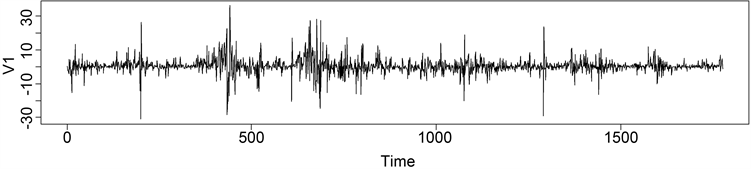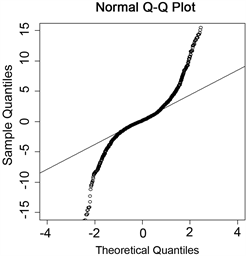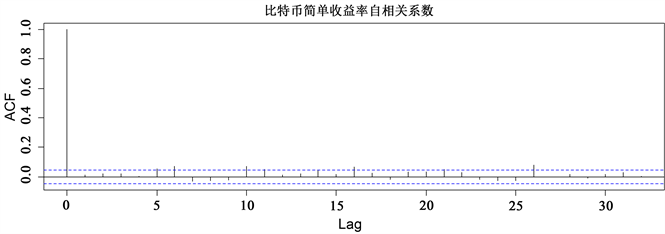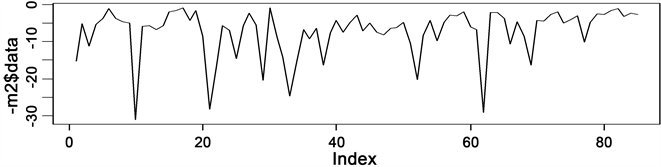# 比特币市场风险度量——基于GARCH-t模型与GEV模型的比较研究A Comparative Study of the RiskMeasurement of the Bitcoin Market Based on the GARCH-T Model and the GEV Model

• 全文下载: PDF(784KB)    PP.19-27   DOI: 10.12677/FIN.2019.91003
• 下载量: 289  浏览量: 772   科研立项经费支持

This paper makes an empirical study on the simple return rate sequence of the special currency with the market data from January 1, 2012 to December 30, 2016, and finds that the sequence has the characteristics of peak, thick tail and fluctuation agglomeration. Using GARCH-t model and EVT model to calculate VaR, it is found that EVT model can better capture the tail characteristics of bitcoin compared with GARCH model, and EVT model can be used to fit the tail distribution of yield, which can more accurately measure bitcoin market risk.

1. 引言

2. 相关模型介绍

2.1. VaR

VaR称为在险价值是由摩根公司首先提出，它显示了金融头寸在一定的持有期，以一定的概率所发生的最大损失  。其本质是收益率分布的分位数，因此定义一个多头头寸在持有期为t，置信度为p的

VaR为 $\text{VaR}p=\mathrm{inf}\left\{x|F\left(x\right)\ge p\right\}$ ，其中inf表示满足 $F\left(x\right)\ge p$ 的最小实数。

$LR=-2\mathrm{ln}\left[{\left(1-p\right)}^{T-N}{p}^{N}\right]+2\mathrm{ln}\left\{\left[1-{\left(N/T\right)}^{T-N}{\left(N/T\right)}^{T}\right]\right\}$

2.2. GARCH模型

1982年恩格尔在研究英国通货膨胀问题时发现时间序列在某些阶段波动持续偏大，在某些阶段又持续小幅波动，这种特征在统计学称为异方差性。为了更好的捕捉这些特征，恩格尔提出了ARCH模型对具有异方差特征的时间序列进行建模。而后波勒斯列夫又在ARCH模型的基础上提出了广义自回归条件异方差模型，以解决ARCH模型在高阶时的参数估计问题。一般GARCH模型可以表示为：

${r}_{t}={\phi }_{o}+\underset{i=1}{\overset{p}{\sum }}{\phi }_{i}{r}_{t-i}+{a}_{t}-\underset{i=1}{\overset{q}{\sum }}{\theta }_{i}{a}_{t-i}$

$\begin{array}{l}at=\sigma t\epsilon t\\ {\sigma }_{t}^{2}=\omega 0+\underset{i=1}{\overset{m}{\sum }}\alpha i{a}_{t-i}^{2}+\underset{j=1}{\overset{s}{\sum }}\beta j{\sigma }_{t-j}^{2}\end{array}$

2.3. 极值理论

$F\left(x\right)=\left\{\begin{array}{c}1-\mathrm{exp}\left[-{\left(1+kx\right)}^{1/k}\right]若k\ne 0\\ 1-\mathrm{exp}\left[-\mathrm{exp}\left(x\right)\right]若k=0\end{array}$

GEV的意义在于不管数据原始分布是什么样子的，其极值的渐进分布都服从广义极值分布。GEV标

$F\left(x\right)=\left\{\begin{array}{c}1-\mathrm{exp}\left[-{\left(1+kx\right)}^{1/k}\right],若x<-1/k\\ 1,其他\end{array}$

$F\left(x\right)=\left\{\begin{array}{c}1-\mathrm{exp}\left[-{\left(1+k\right)}^{1/k}\right],若x>-1/k\\ 0,其他\end{array}$

3. 模型建立及分析

3.1. 数据选取

2009年比特币刚刚提出，市场化程度不高，还不为人所熟知，收益率数据不具有代表性。因此本文选取2012年1月1日至2016年12月30日以美元标价比特币的简单收益率为样本数据的时间序列图，如图1所示，可以看出比特币的简单收益率序列大部分时段呈现平稳态势，但在某些阶段波动持续偏大，某些阶段波动持续偏小。根据时序图初步判断简单收益率序列平稳，波动具有集聚性(数据来源https://cn.investing.com，数据处理采用R3.4.2)。Figure 1. Simple rate of return timing diagram

3.2. 描述性分析

3.2.1. 正态性Figure 2. The Q-Q figure

3.2.2. 平稳性

3.2.3. 自相关性Figure 3. Simple rate of return autocorrelation

3.3. 实证分析

3.3.1. GARCH模型拟合Table 1. Red pool information criteria

$\begin{array}{l}{R}_{t}={\mu }_{t}+{\epsilon }_{t};\\ {\epsilon }_{t}={\sigma }_{t}{e}_{t};\\ {\sigma }_{t}^{2}=\omega +\alpha \left(L\right){\epsilon }_{t}^{2}+\beta \left(L\right){\sigma }_{t}^{2}\end{array}$Table 2. Parameter estimation resultsTable 3. Residual variance test

3.3.2. GEV模型拟合Figure 4. Simple yield minima sequence diagramTable 4. Parameter estimation of generalized extremum distribution

3.3.3. VaR的计算与回测检验Table 5. Backtest results when the backtest sample size is 1700Table 6. Results of backtest when the sample size of backtest is 1500

4. 结论

  王宗润, 吴伟韬, 陈超, 周艳菊. 人民币汇率风险的测度[J]. 统计与决策, 2009(7): 118-120.  林宇, 黄登仕. 胖尾分布及长记忆下的动态EVT-VaR测度研究[J]. 管理科学学报, 2011, 14(7): 71-82.  陈坚. 中国股票市场尾部风险与收益率预测——基于Copula与极值理论的VaR对比研究[J]. 厦门大学学报, 2014(4): 45-54.  戴琳. 比特币投资风险分析[J]. 财会通讯, 2015(5): 3-4.  刘刚, 刘娟. 比特币价格波动与虚拟货币风险防范——基于中美政策信息的事件研究法[J]. 广东财经大学学报, 2015, 30(3): 30-40.  李靖, 徐黎明. 比特币市场风险测度的实证研究[J]. 统计与决策, 2016(5): 161-163.  刘子菲, 史敬. VaR模型比较技术及其评价——理论实证回顾及其应用初探[J]. 金融研究, 2008(5): 130-137.  李靖. 比特币的发展体系研究[J]. 财政金融, 2015(20): 25-28.